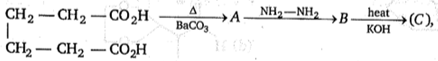Product (C) obtained is :

(a) ${\mathrm{CH}}_{3}-\mathrm{CH}=\mathrm{CH}-{\mathrm{CH}}_{2}$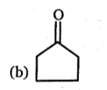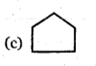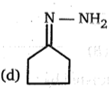Concept Questions :-

Carboxylic Acids
High Yielding Test Series + Question Bank - NEET 2020

Difficulty Level: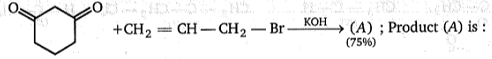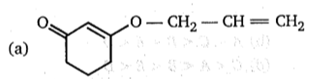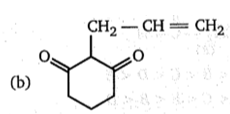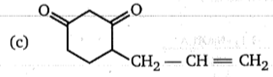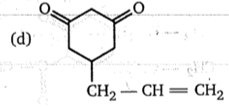Concept Questions :-

Preparation & properties of Aldehydes and Ketones
High Yielding Test Series + Question Bank - NEET 2020

Difficulty Level:

Compound (C) can show geometrical isomerism, Product (E) of the reaction will be :

(a) ${\mathrm{CH}}_{3}-\stackrel{\stackrel{\mathrm{O}}{\parallel }}{\mathrm{C}}-{\mathrm{CH}}_{3}$

(b) ${\mathrm{CH}}_{3}-{\mathrm{CH}}_{2}-\stackrel{\stackrel{\mathrm{O}}{\parallel }}{\mathrm{C}}-\mathrm{H}$

(c) ${\mathrm{CH}}_{3}-\mathrm{CHO}$

(d) HCHO

Concept Questions :-

Preparation & properties of Aldehydes and Ketones
High Yielding Test Series + Question Bank - NEET 2020

Difficulty Level:

Arrange in their increasing order of equilibrium constants for hydraion ?

(a) ${\mathrm{CH}}_{3}-\underset{\left(\mathrm{A}\right)}{\overset{\stackrel{\mathrm{O}}{\parallel }}{\mathrm{C}}}-{\mathrm{CH}}_{3}$,     ${\mathrm{CH}}_{3}-\underset{\left(\mathrm{B}\right)}{\overset{\stackrel{\mathrm{O}}{\parallel }}{\mathrm{C}}}-\mathrm{H}$,

(a) A < B < C < D < E

(b) A < C < B < E < D

(c) A < C < E < B < D

(d) C < A < B < E < D

Concept Questions :-

Nucleophillic Addition, Reactivity of Alpha Hydrogen in Aldehydes with Uses
High Yielding Test Series + Question Bank - NEET 2020

Difficulty Level:

(P); Product (p) of the reaction will be :

(a) $\mathrm{Ph}-{\mathrm{CH}}_{2}-\stackrel{\stackrel{\mathrm{O}}{\parallel }}{\mathrm{C}}-\mathrm{H}$

(b) $\mathrm{Ph}-{\mathrm{CH}}_{2}-\stackrel{\stackrel{\mathrm{O}}{\parallel }}{\mathrm{C}}-{\mathrm{CH}}_{3}$

(c) $\mathrm{Ph}-\mathrm{C}\underset{\stackrel{\text{l}}{{\mathrm{CH}}_{3}}}{\mathrm{H}}-\stackrel{\stackrel{\mathrm{O}}{\parallel }}{\mathrm{C}}-\mathrm{H}$

(d) $\mathrm{Ph}-\mathrm{C}\underset{\stackrel{\text{l}}{{\mathrm{CH}}_{3}}}{\mathrm{H}}-\stackrel{\stackrel{\mathrm{O}}{\parallel }}{\mathrm{C}}-{\mathrm{CH}}_{3}$

Concept Questions :-

Preparation & properties of Aldehydes and Ketones
High Yielding Test Series + Question Bank - NEET 2020

Difficulty Level: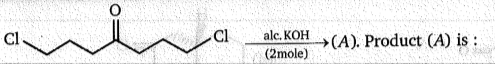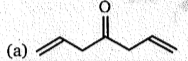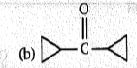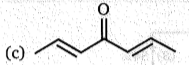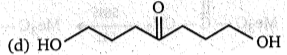Concept Questions :-

Preparation & properties of Aldehydes and Ketones
High Yielding Test Series + Question Bank - NEET 2020

Difficulty Level:

Diastereomers Reactent (A) is :

(a) ${\mathrm{CH}}_{3}-\stackrel{\underset{\parallel }{\mathrm{O}}}{\mathrm{C}}-{\mathrm{CH}}_{3}$

(b) ${\mathrm{CH}}_{3}-\stackrel{\underset{\parallel }{\mathrm{O}}}{\mathrm{C}}-{\mathrm{CH}}_{2}-{\mathrm{CH}}_{3}$
(c) ${\mathrm{CH}}_{3}-{\mathrm{CH}}_{2}-\stackrel{\underset{\parallel }{\mathrm{O}}}{\mathrm{C}}-{\mathrm{CH}}_{2}-{\mathrm{CH}}_{3}$

(d) ${\mathrm{CH}}_{3}-{\mathrm{CH}}_{2}-{\mathrm{CH}}_{2}-\mathrm{CH}=\mathrm{O}$

Concept Questions :-

Preparation & properties of Aldehydes and Ketones
High Yielding Test Series + Question Bank - NEET 2020

Difficulty Level: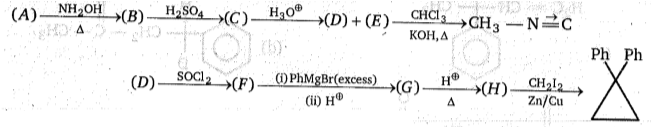Molecular weight of compound (A) is:

(a) 58

(b) 120

(c) 60

(d) 182

Concept Questions :-

Acid Derivatives, Preparation and Properties
High Yielding Test Series + Question Bank - NEET 2020

Difficulty Level: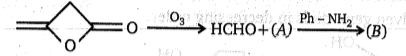Product (B) is:

(a) $\mathrm{Ph}-\mathrm{NH}-\stackrel{\underset{\parallel }{\mathrm{O}}}{\mathrm{C}}-{\mathrm{CO}}_{2}\mathrm{H}$

(b) $\mathrm{Ph}-\mathrm{NH}-\stackrel{\underset{\parallel }{\mathrm{O}}}{\mathrm{C}}-{\mathrm{CH}}_{2}-{\mathrm{CO}}_{2}\mathrm{H}$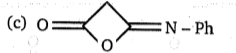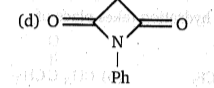Concept Questions :-

Nucleophillic Addition, Reactivity of Alpha Hydrogen in Aldehydes with Uses
High Yielding Test Series + Question Bank - NEET 2020

Difficulty Level: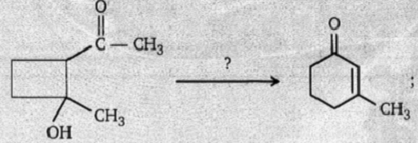This conversion can be achived by :

(a) Dehydration, Hydrolysis

(b) Retro aldol and further condensation

(c) Perkin condensation & Clemmensen reduction

(d) Clemmensen and Perkin condensation

Concept Questions :-

Nucleophillic Addition, Reactivity of Alpha Hydrogen in Aldehydes with Uses